# Sobolev inner product

Let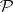be the linear space of polynomials in one variable with real coefficients and let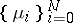be a set of positive Borel measures supported in the real line (cf. also Borel measure; Polynomial).

One introduces an inner product in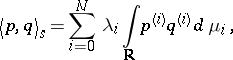(a1)

such that the integrals are convergent for alland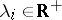. Here,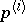is theth derivative of.

As usual, the associated norm isInner products such as (a1) appear in least-square approximation when smooth conditions are involved both in the approximation and in the functions to be approximated. See [a4] for an introduction to this.

One says that (a1) is a Sobolev inner product in.

In a pioneer work, P. Althammer [a1] considered the so-called Legendre–Sobolev inner products, when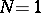and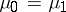is the Lebesgue measure supported on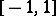. Most of the tools of the standard case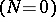are not useful for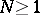since a basic property concerning the symmetry of the shift operator is lost for (a1). This is the reason why further work focused initially on some particular cases of (a1) when.

In [a6], the casethe Gegenbauer weight function and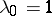is considered with some detail. In such a situation, there exists a linear differential operator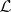of second order such that. This fact leads to the study of the algebraic properties of the so-called Gegenbauer–Sobolev orthogonal polynomials, with a special emphasis on the location of their zeros as well as their strong asymptotics (see [a10]; cf. also Orthogonal polynomials).

A similar approach was made in [a7] for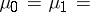the Laguerre weight function and. Thus, the Laguerre–Sobolev orthogonal polynomials are introduced. Some estimates for them, as well as their relative asymptotics with respect to Laguerre polynomials off the positive real semi-axis, are given in [a8].

Beyond these two examples, an approach to a general theory was started in [a3], where the concept of a coherent pair of measures is introduced. The main idea consists in the assumption of a kind of correlation between the measures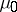and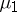.

Consider an inner product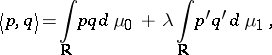(a2)

with, and let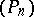andbe sequences of monic polynomials orthogonal with respect toand, respectively.

Thenis called a-coherent pair of measures ifwith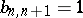and.

If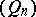denotes the sequence of monic polynomials orthogonal with respect to (a2) andis a-coherent pair, then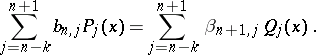Thus, analytic properties ofcan be studied in terms of analytic properties of. The first problem is to classify the set of-coherent pairs of measures. This was described in [a12] for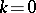(see Table 1). Note that one of the measures must be the Jacobi or the Laguerre weight function. This means that the concept is very restrictive from the point of view of a general theory. The study of the general case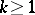remains open (as of 2000).Figure: s130410a

Table 1

Nevertheless, in [a9] a first approach is given whenis the Jacobi weight function.

Let,, supported on. The measureis said to be admissible with respect toif

i)belongs to the Szegö class, i.e.,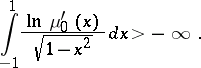ii)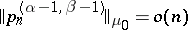,, where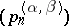denotes the sequence of orthonormal Jacobi polynomials. In such a case one obtains the following relative asymptotics: for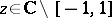,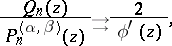where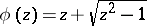, withwhen.

This result has been extended [a11] to the case whenandare absolutely continuous measures supported inand belong to the Szegö class.

In fact,,.

From a numerical point of view, [a2] is a nice survey about the location of zeros of polynomials orthogonal with respect to (a1) when. For more information about Sobolev inner products, see the surveys [a5] and [a13].